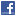## General Mathematics## Tutorial: The Real Powers from Extension of Two Determining Properties of Positive-Integer Powers

Authors: Steven Kenneth Kauffmann

This tutorial parlays two determining properties of positive-integer power functions of a positive real variable into closed formulas for the real power functions of a positive real variable. These two determining properties are that any positive-integer power of unity equals unity, and the linear first-order differential equation that a positive-integer power function of a real positive variable satisfies, which is implicit in its derivative. These two determining properties of positive-integer power functions of a positive real variable are extended to arbitrary real values of the positive-integer power. The extended linear first-order differential equations and initial conditions are then used to generate the Taylor expansions of those real power functions of a positive real variable around the zero value of the real power; this can be carried out in at least two different ways. Those Taylor expansions converge for every real value of the power and every positive real value of the variable, and are readily reexpressed entirely in terms of the exponential function and its inverse; one thus has closed formulas for all the real power functions of a positive real variable. Logarithms describe arbitrary positive numbers as real powers of a given positive number; they can expressed entirely in terms of the exponential function's inverse. The value of the particular positive constant whose powers yield the exponential function itself is worked out.

### Submission history

[v1] 2019-09-22 08:27:44
[v2] 2019-09-26 06:39:58 (removed)
[v3] 2019-09-27 20:16:27 (removed)
[v4] 2019-09-29 20:36:38